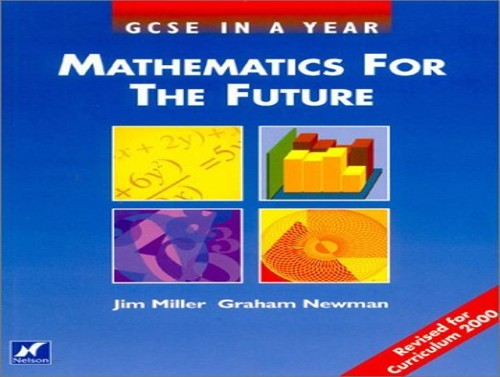# GCSE in a Year by Jim Millin

In Stock
\$11.99
+10% GST
Maths for the Future has been written specifically for students taking a one year GCSE course, whatever syllabus. Pitched at intermediate level, it is ideal for students re-taking their GCSE or for mature students studying GCSE Mathematics for the first time.
8 in stock

## GCSE in a Year Summary

### GCSE in a Year: Mathematics for the Future by Jim Millin

Fully up-to-date with the new 2000 syllabus, this title includes lots of support for self study, including helpful worked examples and guidance in how to present the answers. Including a complete range of past exam papers and examination questions in the style of the latest GCSE exam papers, it helps students to improve their grade through abundant practice questions. The text has been written to be appropriate for older students.

### Why buy from World of BooksOur excellent value books literally don't cost the earthFree delivery in AustraliaEvery used book bought is one saved from landfill

Part 1 Using numbers: mental mathematics; pen and paper methods; calculator methods; number size; indices; equivalent fractions; mixed and improper fractions; cancelling fractions; simple addition and subtraction of fractions; simple multiplication and division of fractions; using fractions; using decimals; using a calculator; rounding; decimal places; significant figures; estimation; standard form; appropriate accuracy; review; ideas for investigations. Part 2 Percentages: percentages; finding percentages; increase and decrease by a percentage; VAT; credit agreements; simple interest; compound interest; wages and salaries; expressing in percentages; inverse percentages; review; ideas for investigations. Part 3 Number relations: ratio; proportional parts; direct proportion; inverse proportion; house insurance; speed; average speed; foreign currencies; directed numbers; number types; squares and cubes; products of primes; review; ideas for investigations. Part 4 Patterns and relationships: use of simple formulas; substituting into a formula; relationships; number patterns; writing formulas for relationships; use of calculators; use of memory; rules of indices; simplifying algebraic expressions; brackets; multiplying brackets; factorizing algebraic expressions; sequences; methods of generating sequences; further sequences; review; ideas for investigations. Part 5 Formulas, equations and inequalities: simple equations; using letters; further equations; useful tips in solving equations; negative and fractional solutions; brackets; problems; solving equations by "trial and improvement"; simultaneous equations; use in problems; manipulating formulas; evaluating formulas; inequalities; two conditions; review; ideas for investigations. Part 6 Graphical representation: coordinates; mappings and plotting graphs; drawing a line from points lying on it; the straight line equation "y=mx+c"; solving simultaneous equations graphically; curves (1) - the quadratic function; curves (2) - the cubic function; curves (3) - the reciprocal function; plotting simple quadratic functions; using quadratic graphs to solve equations; regions; travel graphs; review; ideas for investigations. Part 7 Measures: metric units; imperial units; approximate equivalents; using units; perimeter area; the circle; volume; surface area; direction; a final word on units; review; ideas for investigations. Part 8 Geometry and trigonometry: congruence; angles; triangles; parallel lines; quadrilaterals; solids; nets; accurate drawings; scale drawings; loci; Pythagoras' theorem; trigonomety; review; ideas for investigations. Part 9 Symmetry: reflection; planes of symmetry; rotation; enlargement; regular polygons; tessellations; moving shapes - translation; transformation combinations; review; ideas for investigations. Part 10 Collecting and processing: data collecting sheets; information from tables and lists; ordering data; grouping data; discrete and continuous data; mode and median; mode an

GOR001672136
GCSE in a Year: Mathematics for the Future by Jim Millin
Jim Millin
Used - Very Good
Hardback
Oxford University Press
2000-09-07
1
0174315309
9780174315308
N/A
Book picture is for illustrative purposes only, actual binding, cover or edition may vary.
This is a used book - there is no escaping the fact it has been read by someone else and it will show signs of wear and previous use. Overall we expect it to be in very good condition, but if you are not entirely satisfied please get in touch with us.